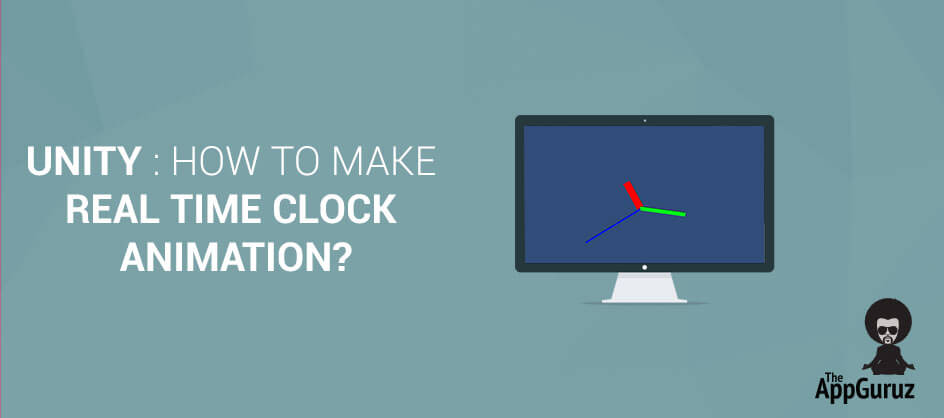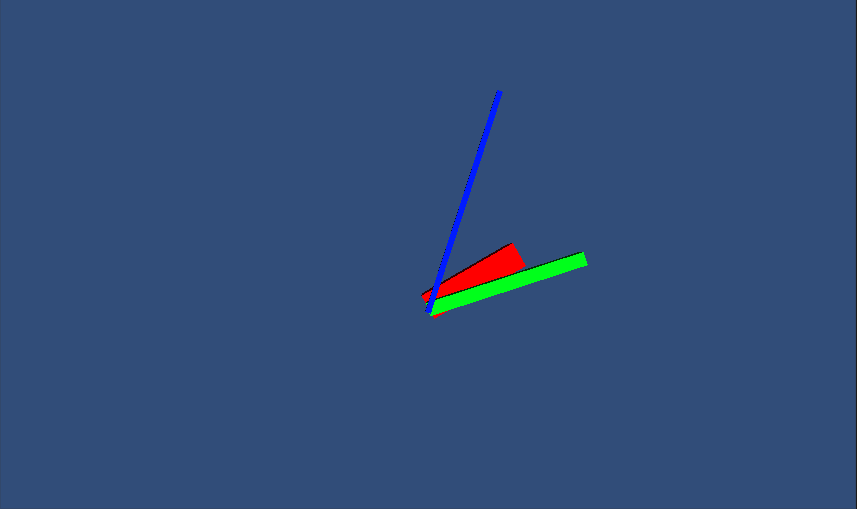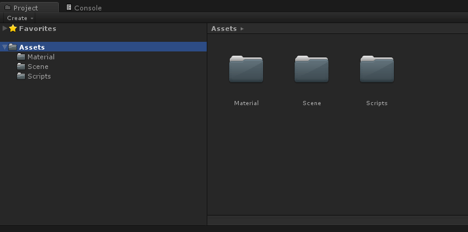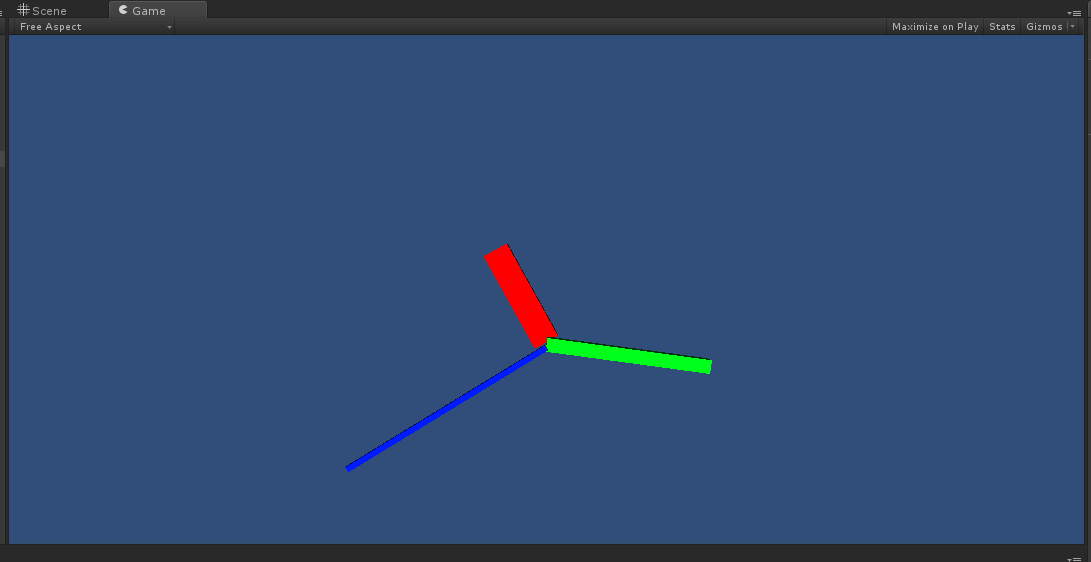## Objective

It's easy to implement. You have to write few lines of code and it's done. In this blog post, we will make two types of clock animation.

Output:1. Digital Type: Show only discrete steps.
2. Analog Type: Smooth flow.

Before I start coding the app, I would like to give you some knowledge about unity terms, which we are going to use here.

What is material?

In unity, a material is used to give detail to an object so we can decide what it will look like. In most cases, material will take textures as parameter.

For Example, if you want to apply brick texture to the wall then drag and drop that texture to material. In this example we are not using any texture. We have used its color property only. We can also give two or more textures to a material.

What is TimeSpan?

Timespan will represent a length of time. We can create or manipulate TimeSpan instances in a C# program.

What is DataTime?

DateTime will give us a date and time in every second. Where Timespan will update in fraction of a second. So it will give us real-time output.

What is Quaternion?

Quaternion's are based on complex numbers and are used to represent 3D rotations. While it is harder to understand than simple 3D vectors, they have some useful characteristics.

What is Quaternion.Euler?

It will return the rotation.
public Quaternion rotation = Quaternion.Euler(0,30,0);

It will rotate z degrees around the z axis, x degrees around the x axis and y degrees around the y axis.

What is Transform.localRotation?

The rotation of the transform is relative to the parent transform's rotation. Whenever you have to rotate an object, which is in child, use this method.

What is the difference between Transform.rotation and Transform.localRotation?

If your GameObject is parented to something then use Transform.localRotation otherwise use simple Transform.rotation. If your GameObject is parented to nothing then both will give you the same output.

## Step 1Basic Project set up.

### 1.1Make a project

• Open Unity.
• Now click on Create New Project.
• Select 3D project.
• And hit Create.
• In the project window creates 3 folders.
• Assets >> Material
• Assets >> Scene
• Assets >> Scripts
• Now save the scene into the Scene Folder.
• Now add Directional light into the scene by clicking right on Hierarchy window and select

Light >> Directional Light.

Project is set up now. Let's start adding some objects into the scene.## Step 2Scene Setup

### 2.1Hierarchy Setup

• First add an empty game object in hierarchy window by clicking right on window and select Create Empty.
• Rename this object to a clock.
• Now in clock object make 3 type of child empty game object. For that just right click on clock object and select Create Empty.
• Now we have to give this game object a meaningful name. Rename it to Hour, Minute, Second.
• Now in each game object we have to create one 3D cube. Right click on each object and select 3D Object >> Cube.

So it will look like this:• Hour > Cube
• Minute > Cube
• Second > Cube
• Make sure all set to 0,0,0.

### 2.2Set the Cube object

• Using this cube, we are displaying clock animation.
• Use below position and scale for each cube.
• First select the particular cube and go to the inspector window and set this property.
 Hour > Cube Position 0,1,0.5 Scale 0.5,2,0.5 Minute > Cube Position 0,1.5,0 Scale 0.25,3,0.25 Second > Cube Position 0,2,-0.5 Scale 0.1,4,0.1

After doing this, it will look like this:### 2.3Set the material for Cube

• For easy understanding, we have to change a color of the cube. So we can easily find out that which cube is for which game object.
• For that, you have to make material and apply to a cube.
• For material first right click on a project then Create >> Material.
• Here we have created 3 materials. Named as Red, Blue, and Green.
• Now in each material we have to set property Main Color.

For:

• Hour >> set Red color
• Minute >> set Green color
• Second >> set Blue color

After doing this, it will look like:

Now in:

• Hour > Cube: Apply Red material.
• Minute > Cube: Apply Green material.
• Second > Cube: Apply Blue material.## Step 3Scripting for animation

### 3.1Create script.

• Go in Assets >> Script and right click and create new c# script.
• Name as ClockAnimation.
• Now double click to open it.

### 3.2Create variables

• First we need the reference of transform to rotate the object.
``public Transform hourTransform, minuteTransform, secondTransform;``
• We need three types of float variables for rotation.
``private float degreesInHour, degreesInMinute, degreesInSecond;  ``
• And one Boolean variable for analog/ digital selection.
``public bool analog;``

### 3.3Start Method

• In Start method we will write following code.
• degreesInHour will indicate that how many degrees will rotate in one hour.
• degreesInMinute will indicate that how many degrees will rotate in one minute.
• degreesInSecond will indicate that how many degrees will rotate in one second.
``````void Start ()
{
degreesInHour = 360 / 12;     // rotate 360/12 in hour.
degreesInMinute = 360 / 60;    // rotate 360/60 in minute.
degreesInSecond = 360 / 60;    // rotate 360/60 in second.
}``````

### 3.4Update Method

Now add this code in the Update method. The update will be called once every frame. So using this we can set clock rotation. Here we need to change the local rotation. We are using Quaternion.Euler to the rotate arm.

``````void Update ()
{
if (analog)
{
TimeSpantimespan = DateTime.Now.TimeOfDay;
hourTransform.localRotation = Quaternion.Euler(0f, 0f, (float)timespan.TotalHours * -    degreesInHour);
minuteTransform.localRotation = Quaternion.Euler(0f, 0f, (float)timespan.TotalMinutes *     -degreesInMinute);
secondTransform.localRotation = Quaternion.Euler(0f, 0f, (float)timespan.TotalSeconds *     -degreesInSecond);
}
else
{
DateTime time = DateTime.Now;
hourTransform.localRotation = Quaternion.Euler(0f, 0f, time.Hour * -degreesInHour);
minuteTransform.localRotation = Quaternion.Euler(0f, 0f, time.Minute * -    degreesInMinute);
secondTransform.localRotation = Quaternion.Euler(0f, 0f, time.Second * -    degreesInSecond);
}
}``````

### 3.5Assign Script

• Now just drag and drop this script to Clock Game object in the hierarchy.
• Assign reference to a script.It's done. Now save the project and run the game. You can see the real time clock. You can check analog for analog movement otherwise it will work as a simple clock.

## Challenges

1. Here we have used Quaternion to rotate the transform but is there any way we can achieve this without using Quaternion?
2. Make Stopwatch using Timespan.
3. Make rotation to anticlockwise.

## Conclusion

So after this we can conclude that using DataTime will give us time in every second where in Timespan we can get a fraction of second so it will work smoothly. If you have time-based game, where you want to display real-time animation then this will be helpful.

If you have any query regarding the project, feel free to contact us.

Got an Idea of Game Development ? What are you still waiting for? Contact us now and see the Idea live soon. Our company has been named as one of the best Unity 3D Game Development Company in IndiaI am professional game developer, developing games in unity (for all platforms). I am very passionate about game development and aim to create addictive, interactive, high quality games.